# 表示量子比特状态

[toc]

## 1.经典比特与量子比特

### 1.1 状态向量

$$x=4$$

$$|x\rangle = \begin{bmatrix} 0\ \vdots \ 0 \ 1 \ 0 \ \vdots \ 0 \end{bmatrix} \begin{matrix} \ \ \ \leftarrow \ \ \ \ \end{matrix} \begin{matrix} \ \ \text{Probability of} \ \text{car being at} \ \text{position 4} \ \ \ \end{matrix}$$

### 1.2量子符号

c = 0

$$|0\rangle = \begin{bmatrix} 1 \ 0 \end{bmatrix} , , , , |1\rangle =\begin{bmatrix} 0 \ 1 \end{bmatrix}.$$

$$|q_0\rangle = \begin{bmatrix} \tfrac{1}{\sqrt{2}} \ \tfrac{i}{\sqrt{2}} \end{bmatrix} .$$

$$|a\rangle = \begin{bmatrix}a_0 \ a_1 \ \vdots \ a_n \end{bmatrix}, \quad |b\rangle = \begin{bmatrix}b_0 \ b_1 \ \vdots \ b_n \end{bmatrix}$$

$$|a\rangle + |b\rangle = \begin{bmatrix}a_0 + b_0 \ a_1 + b_1 \ \vdots \ a_n + b_n \end{bmatrix}$$

$$x|a\rangle = \begin{bmatrix}x \times a_0 \ x \times a_1 \ \vdots \ x \times a_n \end{bmatrix}$$

\begin{aligned} |q_0\rangle & = \tfrac{1}{\sqrt{2}}|0\rangle + \tfrac{i}{\sqrt{2}}|1\rangle \ & = \tfrac{1}{\sqrt{2}}\begin{bmatrix}1\0\end{bmatrix} + \tfrac{i}{\sqrt{2}}\begin{bmatrix}0\1\end{bmatrix}\ & = \begin{bmatrix}\tfrac{1}{\sqrt{2}}\0\end{bmatrix} + \begin{bmatrix}0\\tfrac{i}{\sqrt{2}}\end{bmatrix}\ & = \begin{bmatrix}\tfrac{1}{\sqrt{2}} \ \tfrac{i}{\sqrt{2}} \end{bmatrix}\ \end{aligned}$$|q_0\rangle = \tfrac{1}{\sqrt{2}}|0\rangle + \tfrac{i}{\sqrt{2}}|1\rangle$$

### 1.3使用 Qiskit 探索量子比特

from qiskit import QuantumCircuit, assemble, Aer
from qiskit.visualization import plot_histogram, plot_bloch_vector
from math import sqrt, pi


qc = QuantumCircuit(1) # Create a quantum circuit with one qubit


qc = QuantumCircuit(1)  # Create a quantum circuit with one qubit
initial_state = [0,1]   # Define initial_state as |1>
qc.initialize(initial_state, 0) # Apply initialisation operation to the 0th qubit
qc.draw()  # Let's view our circuit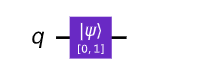sim = Aer.get_backend('aer_simulator')  # Tell Qiskit how to simulate our circuit


qc = QuantumCircuit(1)  # Create a quantum circuit with one qubit
initial_state = [0,1]   # Define initial_state as |1>
qc.initialize(initial_state, 0) # Apply initialisation operation to the 0th qubit
qc.save_statevector()   # Tell simulator to save statevector
qobj = assemble(qc)     # Create a Qobj from the circuit for the simulator to run
result = sim.run(qobj).result() # Do the simulation and return the result


result中，我们可以使用.get_statevector()获得最后的状态向量：

out_state = result.get_statevector()
print(out_state) # Display the output state vector


Statevector([0.+0.j, 1.+0.j],dims=(2,))

qc.measure_all()
qc.draw()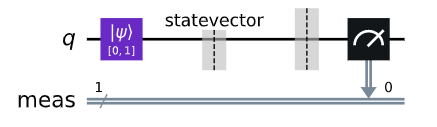qobj = assemble(qc)
result = sim.run(qobj).result()
counts = result.get_counts()
plot_histogram(counts)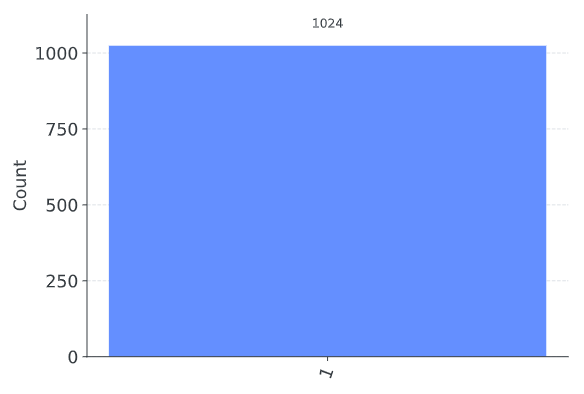$$|q_0\rangle = \tfrac{1}{\sqrt{2}}|0\rangle + \tfrac{i}{\sqrt{2}}|1\rangle$$

initial_state = [1/sqrt(2), 1j/sqrt(2)]  # Define state |q_0>


qc = QuantumCircuit(1) # Must redefine qc
qc.initialize(initial_state, 0) # Initialize the 0th qubit in the state initial_state
qc.save_statevector() # Save statevector
qobj = assemble(qc)
state = sim.run(qobj).result().get_statevector() # Execute the circuit
print(state)           # Print the result


Statevector([0.70710678+0.j, 0.+0.70710678j],dims=(2,))

qobj = assemble(qc)
results = sim.run(qobj).result().get_counts()
plot_histogram(results)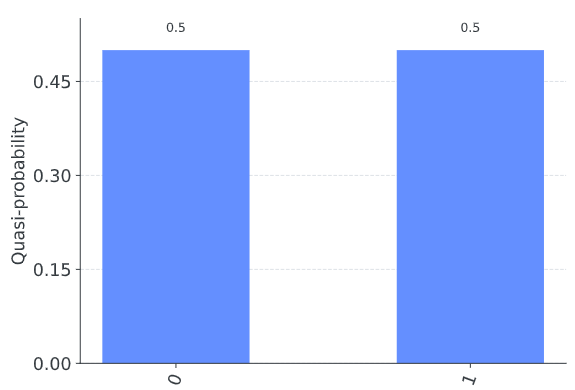## 2.测量规则

### 2.1一个非常重要的规则

$$p(|x\rangle) = | \langle x| \psi \rangle|^2$$

bra-ket之间的转换使用共轭转置方法进行。我们知道 ket（列向量）表示如下:

$$\quad|a\rangle = \begin{bmatrix}a_0 \ a_1 \ \vdots \ a_n \end{bmatrix}$$

$$\langle a| = \begin{bmatrix}a_0^, & a_1^, & \dots & a_n^* \end{bmatrix}$$

$$\langle a| = \begin{bmatrix}a_0^, & a_1^, & \dots & a_n^* \end{bmatrix}, \quad |b\rangle = \begin{bmatrix}b_0 \ b_1 \ \vdots \ b_n \end{bmatrix}$$

$$\langle a|b\rangle = a_0^* b_0 + a_1^* b_1 \dots a_n^* b_n$$

$$\langle1|0\rangle = \begin{bmatrix} 0 & 1\end{bmatrix}\begin{bmatrix}1 \ 0\end{bmatrix} = 0$$

\begin{aligned} \langle0|0\rangle & = \begin{bmatrix} 1 & 0\end{bmatrix}\begin{bmatrix}1 \ 0\end{bmatrix} = 1 \ \langle1|1\rangle & = \begin{bmatrix} 0 & 1\end{bmatrix}\begin{bmatrix}0 \ 1\end{bmatrix} = 1 \end{aligned}

\begin{aligned} |q_0\rangle & = \tfrac{1}{\sqrt{2}}|0\rangle + \tfrac{i}{\sqrt{2}}|1\rangle \ \langle 0| q_0 \rangle & = \tfrac{1}{\sqrt{2}}\langle 0|0\rangle + \tfrac{i}{\sqrt{2}}\langle 0|1\rangle \ & = \tfrac{1}{\sqrt{2}}\cdot 1 + \tfrac{i}{\sqrt{2}} \cdot 0\ & = \tfrac{1}{\sqrt{2}}\ |\langle 0| q_0 \rangle|^2 & = \tfrac{1}{2} \end{aligned}

### #1 规范化

$$\langle\psi|\psi\rangle = 1 \$$

$$|\psi\rangle = \alpha|0\rangle + \beta|1\rangle$$

$$|\alpha|^2 + |\beta|^2 = 1$$

vector = [1,1]
qc.initialize(vector, 0)


QiskitError Traceback (most recent call last)
Input In , in <cell line: 2>()
1 vector = [1,1]
----> 2 qc.initialize(vector, 0)

File /usr/local/lib/python3.8/site-packages/qiskit/extensions/quantum_initializer/initializer.py:191, in initialize(self, params, qubits)
188 qubits = [qubits]
189 num_qubits = len(qubits) if isinstance(params, int) else None
→ 191 return self.append(Initialize(params, num_qubits), qubits)

File /usr/local/lib/python3.8/site-packages/qiskit/extensions/quantum_initializer/initializer.py:57, in Initialize.init(self, params, num_qubits)
36 def init(self, params, num_qubits=None):
37 r""“Create new initialize composite.
38
39 Args:
(…)
55 and the remaining 3 qubits to be initialized to :math:|0\rangle.
56 “””
—> 57 self._stateprep = StatePreparation(params, num_qubits)
59 super().init(“initialize”, self._stateprep.num_qubits, 0, self._stateprep.params)

File /usr/local/lib/python3.8/site-packages/qiskit/circuit/library/data_preparation/state_preparation.py:99, in StatePreparation.init(self, params, num_qubits, inverse, label)
96 self._from_label = isinstance(params, str)
97 self._from_int = isinstance(params, int)
—> 99 num_qubits = self._get_num_qubits(num_qubits, params)
101 params = [params] if isinstance(params, int) else params
103 super().init(self._name, num_qubits, params, label=self._label)

File /usr/local/lib/python3.8/site-packages/qiskit/circuit/library/data_preparation/state_preparation.py:202, in StatePreparation._get_num_qubits(self, num_qubits, params)
200 # Check if probabilities (amplitudes squared) sum to 1
201 if not math.isclose(sum(np.absolute(params) ** 2), 1.0, abs_tol=_EPS):
→ 202 raise QiskitError(“Sum of amplitudes-squared does not equal one.”)
204 num_qubits = int(num_qubits)
205 return num_qubits

QiskitError: ‘Sum of amplitudes-squared does not equal one.’

1. 创建一个状态向量，该向量将给出$1/3$测量概率$|0⟩$。

2. 创建一个不同的状态向量，该向量将给出相同的测量概率。

3. 验证测量的概率$|1⟩$对于这两种状态是$2/3$。

# Run the code in this cell to interact with the widget
from qiskit_textbook.widgets import state_vector_exercise
state_vector_exercise(target=1/3)


### #3 全局相图

$$\begin{bmatrix}0 \ i\end{bmatrix} = i|1\rangle.$$

$$|\langle x| (i|1\rangle) |^2 = | i \langle x|1\rangle|^2 = |\langle x|1\rangle|^2$$

$$|\langle x| ( \gamma |a\rangle) |^2 = | \gamma \langle x|a\rangle|^2 = |\langle x|a\rangle|^2$$

### #4 观察者效应

$$|q\rangle = \alpha|0\rangle + \beta|1\rangle$$

$$|q\rangle = \begin{bmatrix} \alpha \ \beta \end{bmatrix} \xrightarrow{\text{Measure }|0\rangle} |q\rangle = |0\rangle = \begin{bmatrix} 1 \ 0 \end{bmatrix}$$

qc = QuantumCircuit(1) # We are redefining qc
initial_state = [0.+1.j/sqrt(2),1/sqrt(2)+0.j]
qc.initialize(initial_state, 0)
qc.draw()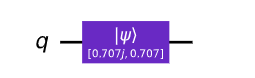$$|q\rangle = \tfrac{i}{\sqrt{2}}|0\rangle + \tfrac{1}{\sqrt{2}}|1\rangle$$

qc.save_statevector()
result = sim.run(assemble(qc)).result()
state = result.get_statevector()
print("Qubit State = " + str(state))


qc = QuantumCircuit(1) # We are redefining qc
initial_state = [0.+1.j/sqrt(2),1/sqrt(2)+0.j]
qc.initialize(initial_state, 0)
qc.measure_all()
qc.save_statevector()
qc.draw()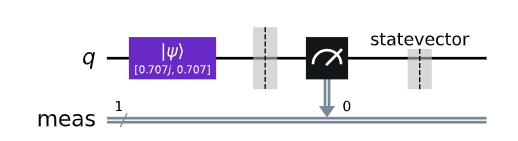qobj = assemble(qc)
state = sim.run(qobj).result().get_statevector()
print("State of Measured Qubit = " + str(state))


#### 关于量子模拟器的说明

$$0110111110$$

## 3.布洛赫球

### 3.1 描述受限量子比特状态

$$|q\rangle = \alpha|0\rangle + \beta|1\rangle$$
$$\alpha, \beta \in \mathbb{C}$$
(第二行告诉我们$α$和$β$是复数)。第2节中的前两个含义告诉我们，我们无法区分其中一些状态。这意味着我们可以更具体地描述量子比特。

$$|q\rangle = \alpha|0\rangle + e^{i\phi}\beta|1\rangle$$
$$\alpha, \beta, \phi \in \mathbb{R}$$

$$\sqrt{\alpha^2 + \beta^2} = 1$$

$$\sqrt{\sin^2{x} + \cos^2{x}} = 1$$

$$\alpha = \cos{\tfrac{\theta}{2}}, \quad \beta=\sin{\tfrac{\theta}{2}}$$

$$|q\rangle = \cos{\tfrac{\theta}{2}}|0\rangle + e^{i\phi}\sin{\tfrac{\theta}{2}}|1\rangle$$
$$\theta, \phi \in \mathbb{R}$$

### 3.2 直观表示量子比特状态

$$|q\rangle = \cos{\tfrac{\theta}{2}}|0\rangle + e^{i\phi}\sin{\tfrac{\theta}{2}}|1\rangle$$

Qiskit有一个绘制布洛赫球的函数plot_bloch_vector()。这个函数默认接受笛卡儿坐标，但也可以通过传递参数coord_type='spherical'接受球坐标。

from qiskit.visualization import plot_bloch_vector
coords = [1,pi/2,0]  # [Radius, Theta, Phi]
plot_bloch_vector(coords, coord_type='spherical')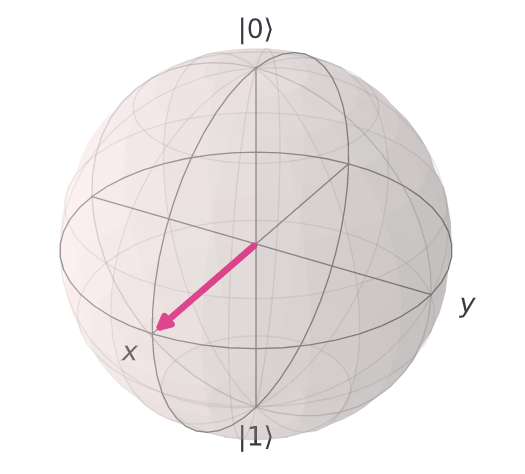#### 快速练习

1.$|0⟩$

2.$|1⟩$

3.$\tfrac{1}{\sqrt{2}}(|0\rangle + |1\rangle)$

4.$\tfrac{1}{\sqrt{2}}(|0\rangle - i|1\rangle)$

5.$\tfrac{1}{\sqrt{2}}\begin{bmatrix}i\1\end{bmatrix}$

import qiskit.tools.jupyter
%qiskit_version_table


### 版本信息

Qiskit Software Version
qiskit-terra 0.22.0
qiskit-aer 0.11.0
qiskit-ibmq-provider 0.19.2
qiskit 0.39.0
qiskit-nature 0.4.1
qiskit-finance 0.3.2
qiskit-optimization 0.4.0
qiskit-machine-learning 0.4.0
System information
Python version 3.8.13
Python compiler Clang 13.1.6 (clang-1316.0.21.2.5)
Python build default, Aug 29 2022 05:17:23
OS Darwin
CPUs 8
Memory (Gb) 32.0